This handy Math in Focus Grade 3 Workbook Answer Key Chapter 16 Practice 3 Addition of Time provides detailed solutions for the textbook questions.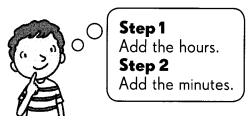Example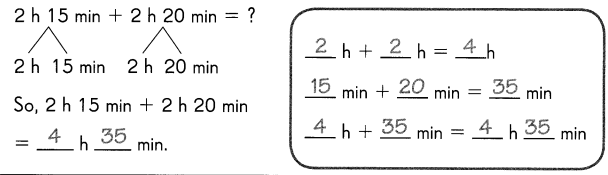Question 1.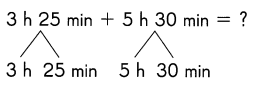So, 3 h 25 min + 5 h 30 min
= ______ h _______ min.

______ h + ______ h = ______ h
______ min + ______ min = ______ min
______ h + ______ min = ______ h ______ min
3. If the minutes are 60 or more, subtract 60 from the minutes and add 1 to hours
Explanation:
= 3 hours+5 hours
=8 hours.
= 25 min+30 min
= 55 min.
Finally, we get in hours and minutes are 8 hours 55 minutes.

Question 2.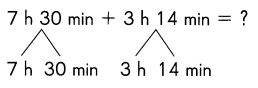So, 7 h 30 min + 3 h 14 min
= ______ h _______ min.

______ h + ______ h = ______ h
______ min + ______ min = ______ min
______ h + ______ min = ______ h ______ min
3. If the minutes are 60 or more, subtract 60 from the minutes and add 1 to hours
Explanation:
= 7 hours+3 hours
=10 hours.
= 30 min+14 min
= 44 min.
Finally, we get in hours and minutes are 10 hours 44 minutes.

Question 3.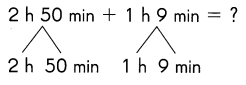So, 2 h 50 min + 1 h 9 min
= ______ h _______ min.

______ h + ______ h = ______ h
______ min + ______ min = ______ min
______ h + ______ min = ______ h ______ min
3. If the minutes are 60 or more, subtract 60 from the minutes and add 1 to hours
Explanation:
= 2 hours+1 hours
=3 hours.
= 50 min+9 min
= 59 min.
Finally, we get in hours and minutes are 3 hours 59 minutes.Question 4.
20 min + 55 min = __________ min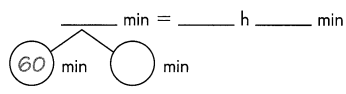Explanation: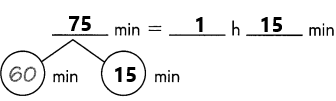The given question is 20 min+55 min=X min.
If we add 20 min and 55 min then we get 20+55=75 min.
To this 75 minutes, we need to convert in hours and minutes.
75 can be written as 60 and 15:
1 hour=60 minute.
And remaining 15 minutes will stay the same.
Finally, 75 min=1 hours 15 minutes.

Question 5.
55 min + 45 min = __________ min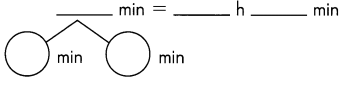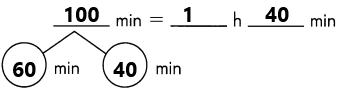The given question is 55 min+45 min=X min.
If we add 55 min and 45 min then we get 55+45=100 min.
To this 100 minutes, we need to convert in hours and minutes.
100 can be written as 60 and 40:
1 hour=60 minute.
And remaining 40 minutes will stay the same.
Finally, 100 min=1 hours 40 minutes.

Question 6.
4 h 46 min + 2 h 14 min = _____ h _____ min
= ________ h
3. If the minutes are 60 or more, subtract 60 from the minutes and add 1 to hours
Explanation:
= 4 hours+2 hours
=6 hours.
= 46 min+14 min
= 60 min.
Step 3: Apply rule 3. We got 60 minutes. So subtract 60 and add 1 to the hours.
=60-60
=0.
= 6 hours+1
=7 hours.
Therefore,  the answer is 7 hours.

Question 7.
1 h 48 min + 3 h 35 min = _____ h _____ min
= _____ h _____ min
3. If the minutes are 60 or more, subtract 60 from the minutes and add 1 to hours
Explanation:
= 1 hours+3 hour
=4 hours.
= 48 min+35 min
= 83 min.
Step 3: Apply rule 3. We got 60 minutes. So subtract 60 and add 1 to the hours.
=83-60
=23.
= 4 hours+1
=5 hours.
Therefore,  the answer is 5 hours 23 minutes.

Solve.

Question 8.
Grace spends 50 minutes practicing the piano. Then she spends 2 hours 1 5 minutes doing her homework. How long does she spend on the two tasks?
The minutes she spends on the piano=50 min
The time spends on her homework=2 h 15 min
The total time she spends on both works=X
By following some steps we need to calculate the total hours and min.
3. If the minutes are 60 or more, subtract 60 from the minutes and add 1 to hours
Explanation: